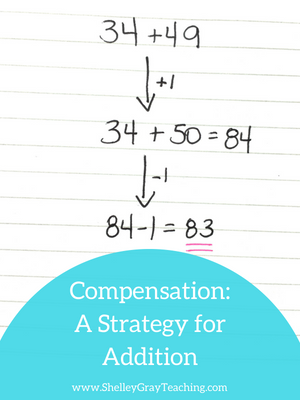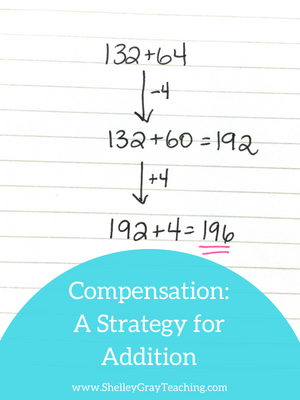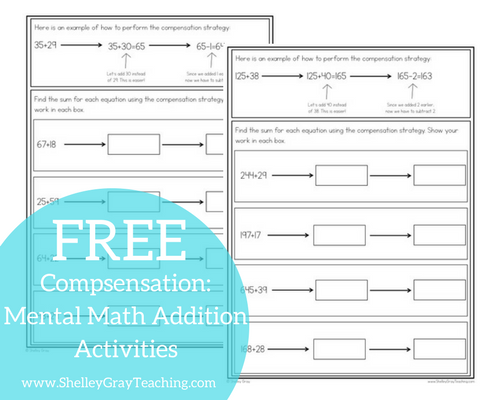Compensation is a mental math strategy for multi-digit addition that involves adjusting one of the addends to make the equation easier to solve. Some students may prefer this strategy as an alternative to left-to-right addition or the breaking up the second number strategy.

Compensation is a useful strategy for making equations easier to solve. More importantly, it encourages students to think flexibly about numbers.

HOW TO PERFORM THE COMPENSATION STRATEGY

Let’s solve the equation 34+49 using the compensation strategy.

First, since 49 is so close to 50, we will add 34+50. This is easier to solve. Then, since we added one extra to the original equation, we have to subtract one from the final answer.Let’s suppose that we want to solve the equation 132+64. We can use compensation to add these two numbers. With compensation, there is no one right way to perform the strategy. In this case, let’s begin by taking 4 away from the 64 and add 132+60 to make 192. This is an easy equation to solve. Now, since we subtracted 4 from the original equation, we have to add 4 to the answer. 192+4=196.NEXT STEPS:

• If you would like full support for teaching addition strategies in your classroom, check out The Addition Station HERE.  These Math Stations are self-paced, student-centered stations for the basic math strategies. Students move through the levels at their own pace, ensuring that they are always challenged, and working to their full potential.